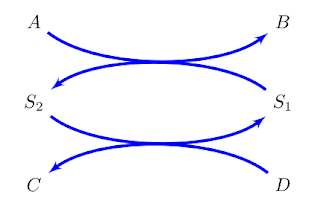## Wednesday, May 4, 2011

### Drawing Pathways with TikZ: Part 1

May 4, 2011 9:45 am

Drawing biochemical pathway diagrams has never been easy. Many of us probably use a combination of Power Point, illustrator, Inkscape, or even MS Paint. Of these Illustrator and Inkscape are probably the most useful but Illustrator is expensive and Inkscape is sometimes a little buggy. Power Point is of course a favorite because it's used so often, perhaps too often in our everyday work. In using these tools we are also forced to maintain separate files from our LaTeX document and in formats that are not related to LaTeX itself.

Although there is an initial learning curve, the LaTeX extension, PGF/TikZ is an alternative to drawing pathway diagrams. Timo Maarleveld, who is doing a graduate project at UW, has played around with TikZ and inspired me to take a second look at TikZ. Last year one of my students published an article on converting SBML to TikZ (www.SBML2Tikz.org) but that approach used absolute coordinates to layout the network. In the following example I have a very simple cycle that is drawn using TikZ that uses some relative positioning though the intersection of the curves is still done using an absolute distance.


\begin{tikzpicture}[>=latex',node distance = 2cm]
\node (S1) {$S_1$};
\node [left of = S1] (S2) {$S_2$};
\node [above of = S2,node distance=1.04cm] (A) {$A$};
\node [above of = S1,node distance=1.04cm] (B) {$B$};
\node [below of = S2,node distance=1.04cm] (C) {$C$};
\node [below of = S1,node distance=1.04cm] (D) {$D$};

\draw [->,thick] (S1) to[bend left=40] node {} (S2);
\draw [->,thick] (S2) to[bend left=40] node {} (S1);
\draw [->,thick] (A) to[bend right=40] node {} (B);
\draw [->,thick] (D) to[bend right=40] node {} (C);
\end{tikzpicture}I wasn't particularly satisfied with this solution mainly because I use absolute dimensions (The 1.04cm) to make sure that the curves intersected at the midpoint. I therefore tried tex.stackexchange where I got the following answer from Dmitry F. Volosnykh:
\documentclass{minimal}

\usepackage{tikz}
\usetikzlibrary{arrows,positioning}

\def \coeffx{0.29}
\def \coeffy{0.91}
\def \nodedistancex{4cm}
\def \nodedistancey{1cm}
\def \offsetx{\nodedistancex*\coeffx}
\def \offsety{\nodedistancey*\coeffy}

\begin{document}

\begin{tikzpicture}[>=latex', node distance=\nodedistancey and \nodedistancex]
\node (S1) {$S_1$};
\node[left=of S1] (S2) {$S_2$};
\node[above=of S2] (A) {$A$};
\node[above=of S1] (B) {$B$};
\node[below=of S2] (C) {$C$};
\node[below=of S1] (D) {$D$};

\draw[->,thick] (S1) .. controls +(-\offsetx,\offsety) and +(\offsetx,\offsety) .. (S2);
\draw[->,thick] (S2) .. controls +(\offsetx,-\offsety) and +(-\offsetx,-\offsety) .. (S1);
\draw[->,thick] (A) .. controls +(\offsetx,-\offsety) and +(-\offsetx,-\offsety) .. (B);
\draw[->,thick] (D) .. controls +(-\offsetx,\offsety) and +(\offsetx,\offsety) .. (C);
\end{tikzpicture}

\end{document}
There was an additional answer from Frédéric which you can see form this link.